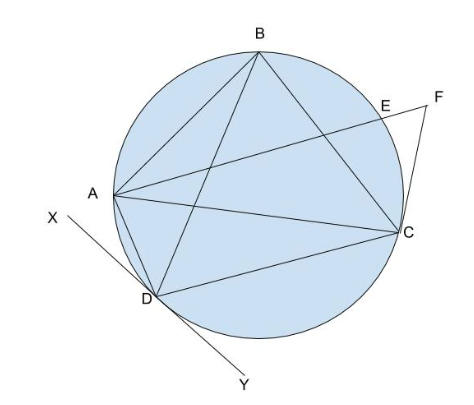# Faisal and His PlaygroundsReplay of NSU Cybernauts...
Limits 1s, 512 MB

Faisal is a famous ice hockey player. He plays his game all across the world. But his favorite ground is Nirpur's Yellow Cat Stadium. Whenever he plays at this ground, he performs. The Yellow Cat Stadium can be considered as a big circle. The ground is a quadrangle inscribed inside the circle, where the four vertices of the ground lies at the circumference of the stadium.In the picture above, the quadrangle $ABCD$ is the area of the ground inside the Yellow Cat Stadium.

Due to the increasing temperature in the City of Dahaka, Yellow Cat Stadium (YCS) is no longer suitable for ice hockey. Thus, Faisal decides to create two grounds by himself, so that he can practice on these two grounds for his upcoming tournaments. Since YCS is his favorite, he wants to create two grounds which are rectangular. The area of them are as follows:

1. The area of the first ground is $AB \times DC$
2. The area of the second ground is $AD \times BC$.

In order to find the area of two rectangles, Faisal has appointed Preplanner, a renowned company that will help to get these two grounds ready. He has given the following information of YCS on the picture(Figure 1) above to that company:

In figure 1, There is a line segment with one endpoint $A$, on the circle, one endpoint $F$ outside the circle and one point between these two points intersecting the circle on point $E$. There will be another line from the external point $F$ that touches another point $C$ that is on the circumference of the circle. The company will be given the length of $CF$, $AD$ and $EF$. There is another line $XY$, that touches the circle on point $D$. The company will be given three angles $BDY$, $AFC$ and $ABD$.

You are a new employee of Preplanner. Your boss has given you the task of finding the summation of the area of two of the grounds that Faisal is going to create.

## Input

The first line contains an integer $T$ ($1 ≤ T ≤ 10^5$), denoting the number of testcases.

The first line of each case contains three real numbers, representing the lengths of $AD$, $CF$ and $EF$. All lengths are less than $10^8$.

The next line will have three real numbers, representing the angles $ABD$, $BDY$ and $AFC$. All the angles will be valid and will be given in degrees and less than $180 \degree$.

## Output

Output a single number, the summation of the areas of the two grounds. The answer should be accurate up to 2 decimal places.

## Sample

InputOutput
1
8 5 2.23606797749979
53.130102354156 90 63.434948822922

100Geometry

### Submit